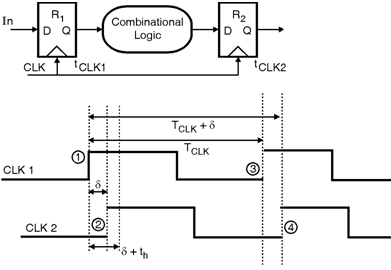Home > System On Chip > Clock Distribution > Clock Skew

# Clock Skew

Clock skew is defined as the variations in the arrival time of clock transition in an integrated circuit. The clock skew between two points x and y in a semicoductor IC is given by  (x,y) = tx- ty, where tx and ty are the position of rising edge of the clock with respect to a reference. Consider the data transfer between the registers R1 and R2. In this system, the routing direction decides whether the clock skew is positive or negative. Figure shows the timing diagram for the positive skew. From figure it can be observed that, the clock edge is delayed by a positive  at the second register. Clock skew is mainly because of static mismatches in the clock. i.e. if one cycle CLK2 lagged CLK1 by , then in the next cycle will lag by the same amount.Fig_Clock Skew